• 6轴机械臂 3D打印资料 3D打印图纸 6轴机械臂——AR2.zip
• 6轴机械臂正逆解运算实现 利用Gluon-6L3机械臂模型的参数（参数来源于知乎@梁政），对机械臂进行运动学分析。 这里采用标准DH坐标系，并将d6设置为0，方便后续计算。 首先，SDH的变换矩阵为： ii−1T=Ai=^{i-1}_iT=A...
6轴机械臂正逆解运算实现
利用Gluon-6L3机械臂模型的参数，对机械臂进行运动学分析。这里采用标准DH坐标系，并将d6设置为0，方便后续计算。
首先，SDH的变换矩阵为：
$^{i-1}_iT=A_i=$$\begin{bmatrix} c_i & -s_ic\alpha_i & s_is\alpha_i& a_ic_i \\ s_i & c_ic\alpha_i & -c_is\alpha_i& a_is_i\\ 0 & s\alpha_i & c\alpha_i&d_i\\ 0&0&0&1\end{bmatrix}$
我们将机械臂参数表中的数据带入，得到6个变换矩阵
$^{0}_1T=$$\begin{bmatrix} c_1& 0 & s_1 &0\\ s_1 & 0 & -c_1 & 0\\ 0 & 1 & 0 &d_1\\ 0 &0 &0 &1 \end{bmatrix}$       $^{1}_2T=$$\begin{bmatrix} c_2& -s_2 & 0 &a_2c_2\\ s_2 & c_2 & 0 & a_2s_2\\ 0 & 0 & 1 &0\\ 0 &0 &0 &1 \end{bmatrix}$
$^{2}_3T=$$\begin{bmatrix} c\theta_3& -s\theta_3 & 0 &a_3c\theta_3\\ s\theta_3 & c\theta_3 & 0 & a_3s\theta_3\\ 0 & 0 & 1 &0\\ 0 &0 &0 &1 \end{bmatrix}$    $^{3}_4T=$$\begin{bmatrix} c\theta_4& 0 & s\theta_4 &0\\ s\theta_4 & 0 & -c\theta_4 & 0\\ 0 & 1 & 0 &d_4\\ 0 &0 &0 &1 \end{bmatrix}$
$^{4}_5T=$$\begin{bmatrix} c\theta_5& 0 & -s\theta_5 &0\\ s\theta_5 & 0 & c\theta_5 & 0\\ 0 & -1 & 0 &d_5\\ 0 &0 &0 &1 \end{bmatrix}$   $^{5}_6T=$$\begin{bmatrix} c\theta_6& -s\theta_6 & 0 &0\\ s\theta_6 & c\theta_6 & 0 & 0\\ 0 & 0 & 1 &0\\ 0 &0 &0 &1 \end{bmatrix}$
机械臂的位姿矩阵T为：
$T=^{0}_1T^{1}_2T^{2}_3T^{3}_4T^{4}_5T^{5}_6T=$$\begin{bmatrix} n_x& o_x & a_x &p_x\\ n_y & o_y& a_y & p_y \\ n_z & o_z & a_z & p_z \\ 0 &0 &0 &1 \end{bmatrix}$
$n_x=c_1c_5c_6c_{234}-c_1s_6s_{234}+s_1s_5s_6$
$n_y=s_1c_5c_6c_{234}-c_1c_6c_{6}-s_1s_5s_{234}$
$n_z=c_5c_6s_{234}+s_6c_{234}$
$o_x=-c_1c_5s_6c_{234}-s_1s_5s_6-c_1c_6s_{234}$
$o_y=-s_1c_5s_6c_{234}+c_1c_5s_6-s_1c_6s_{234}$
$o_z=c_6c_{234}-s_6c_5s_{234}$
$a_x=c_5s_1-c_1c_5c_{234}$
$a_y=-c_5c_1-s_1s_5c_{234}$
$a_z=-s_5s_{234}$
$p_x=c_1d_5s_{234}+s_1d_4+a_3c_1c_{23}+a_2c_2c_1$
$p_y=s_1d_5s_{234}-c_1d_4+a_3s_1c_{23}+a_2c_2s_1$
$p_z=-c_{234}d_5+a_3s_{23}+a_2s_2+d_1$
运动学正解
Euler Angles-由T推算按angles
	bata = atan2(sqrt(pow(T.matric, 2) + pow(T.matric, 2)), T.matric);
alpha = atan2(T.matric / sin(bata), T.matric / sin(bata));
gama = atan2(T.matric / sin(bata), -T.matric / sin(bata));

运动学逆解
将末端位姿输入，反求T中的元素
CaculateThta(double x, double y, double z, double alpha, double bata, double gama)

根据末端位姿写出的T矩阵
（根据台大机器人运动学讲解P11，P12写的T,详细了解https://www.bilibili.com/video/BV1v4411H7ez）
$T=\begin{bmatrix} c_\alpha c_\beta c_\gamma-s_\alpha s_\gamma& -c_\alpha c_\beta s_\gamma-s_\alpha c_\gamma &c_\alpha s_\beta &p_x\\ s_\alpha c_\beta c_\gamma+c_\alpha s_\gamma& -s_\alpha c_\beta c_\gamma+c_\alpha c_\gamma& s_\alpha s_\beta & p_y \\ -s_\beta c_\gamma& s_\beta s_\gamma &c_\beta & p_z \\ 0 &0 &0 &1 \end{bmatrix}$ =$\begin{bmatrix} n_x& o_x & a_x &p_x\\ n_y & o_y& a_y & p_y \\ n_z & o_z & a_z & p_z \\ 0 &0 &0 &1 \end{bmatrix}$
首先，我们对矩阵  $^{0}_1T$进行逆变换
$^{0}_1T^{-1}T=^{1}_2T^{2}_3T^{3}_4T^{4}_5T^{5}_6T=\begin{bmatrix} c_5c_6c_{234}-s_6s_{234}& -c_5s_6c_{234}-c_6s_{234} & -s_5c_{234} &d_5s_{234}+a_3c_{23}+a_2c_2\\ c_5c_6s_{234}-s_6c_{234}& -c_5s_6s_{234}+c_6c_{234} & -s_5s_{234} &-d_5c_{234}+a_3s_{23}+a_2s_2\\ c_6s_5 &-s_6s_5 & c_5 &d_4\\ 0 &0 &0 &1 \end{bmatrix}=$ $\begin{bmatrix} c_1n_x+s_1n_y& c_1o_x+s_1o_y & c_1a_x+s_1a_y &c_1p_x+s_1p_y\\ n_z & o_z& a_z & p_y-d_1 \\ s_1n_x-c_1n_y& s_1o_x-c_1o_y & s_1a_x-c_1a_y &s_1p_x-c_1p_y\\ 0 &0 &0 &1 \end{bmatrix}$
到这一步某些$\theta$角已经现形了，这里计算尽量统一位atan2。
计算$\theta_1$
$d_4=s_1p_x-c_1p_y$  (1)
令$p_x=cos\phi,p_y=sin\phi$带入(1)得
$sin(\theta_1-\phi)=d_4$
$cos(\theta_1-\phi)=\pm\sqrt{1-d_4^2} \quad$
$\theta_1=atan2(p_y,p_x)+atan2(d_4,\pm\sqrt{1-d_4^2} \quad)$
计算$\theta_{5}$
已知$c_{5}=s_1a_x-c_1a_y$
$s_{5}=\pm\sqrt{1-c_5^2} \quad$
$\theta_{5}=atan2(s_{5},c_{5})$
计算$\theta_6$
$c_6s_5=s_1n_x-c_1n_y$
$-s_6s_5=s_1o_x-c_1o_y$
$\theta_6=atan2(\frac{(s_1n_x-c_1n_y)}{s_5} \quad,\frac{(s_1o_x-c_1o_y)}{-s_5} \quad)$
计算$\theta_3$
已知$s_{234}=\frac{a_z}{-s_5} \quad,c_{234}=\frac{c_1a_x+s_1a_y}{-s_5} \quad$（2）
已知$d_5s_{234}+a_3c_{23}+a_2c_2=c_1p_x+s_1p_y$（3）
已知$-d_5c_{234}+a_3s_{23}+a_2s_2=p_z-d_1$（4）
令$k_1=c_1p_x+s_1p_y-d_5s_{234},k_2=p_z-d_1+d_5c_{234}$
$c_3=\frac{k_1^2+k_2^2-a_2^2-a_3^3}{2a_2a_3} \quad$
$s_3=\pm\sqrt{1-c_3^2} \quad$
$\theta_{3}=atan2(s_{3},c_{3})$
计算$\theta_2$
继续利用上面三个公式（2）（3）（4）求解$\theta_2$
$s_2=\frac{(k_2(a_2+a_3c_3)-k_1a_3s_3)}{a_1^2+a_2^2+2a_1a_2c_3} \quad$
$c_2=\frac{k_2-s_2(a_2+a_3c_3)}{a_3s_3} \quad$
$\theta_{2}=atan2(s_{2},c_{2})$
计算$\theta_4$
$s_{234}=s_4c_{23}+s_{23}c_4$
$c_{234}=c_4c_{23}-s_{23}s_4$
两式联立得
$s_4=c_{23}s_{234}-s_{23}c_{234}$
$c_4=\frac{c_{234}+s_{4}s_{23}}{c_{23}} \quad$
$\theta_{4}=atan2(s_{4},c_{4})$
至此，六个角全部求完，根据上式子的表达一共八组解


展开全文• 首先第一步，我们选定一个球形的工件第二步，确定开始时机械臂末端姿态：六末端面垂直向下第一步：ry的讨论我们知道，机械臂末端状态有六个，分别是x,y,z,rx,ry,rz。下面先以ry为基础进行讨论，ry顾名思义，就是...
给出加工工件上的各个点以及各个点所对应的位姿，如何转换为机械臂识别的末端位姿？首先第一步，我们选定一个球形的工件第二步，确定开始时机械臂末端姿态：六轴末端面垂直向下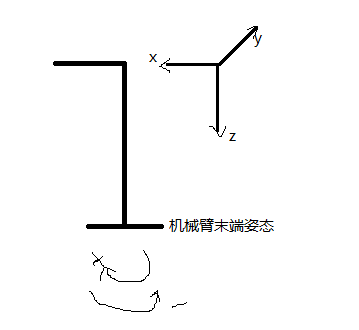第一步：ry的讨论我们知道，机械臂末端状态有六个，分别是x,y,z,rx,ry,rz。下面先以ry为基础进行讨论，ry顾名思义，就是机械臂末端绕y轴旋转的角度，我们通过右手定则可以确定旋转的正负方向。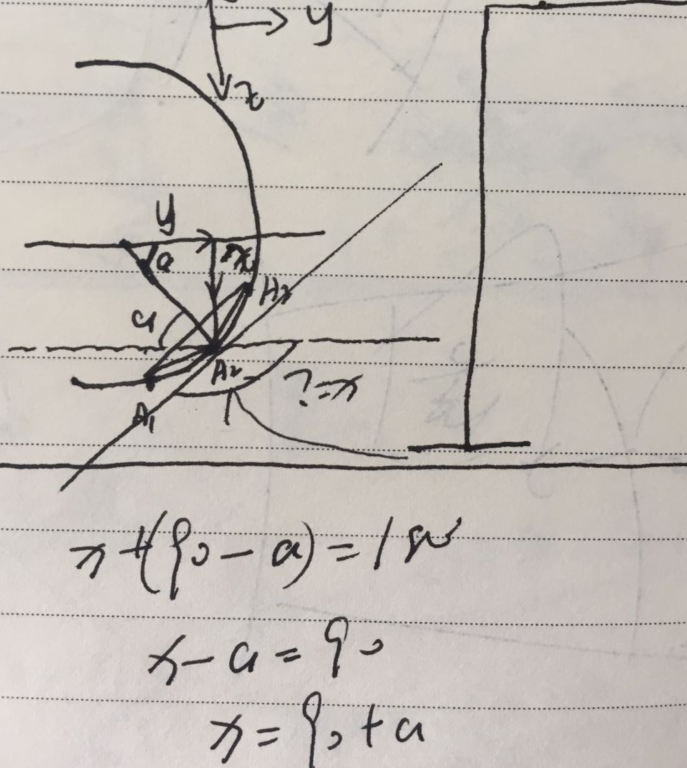上图求出的x即是我们想要的结果，有图可知，机械臂末端旋转方向为负，所以讲得出的结果加上负号即-x,就等于ry第二步：rz的讨论rz的运算与ry类似，这里补充一点，我们使用的方法是将一个球体沿着水平面切出多条密集的线，然后再我们需要计算的点左右各取一个点，通过三点得出该三点的外接圆，然后通过一定的算法得出结果。具体细的不做讨论，可以私聊我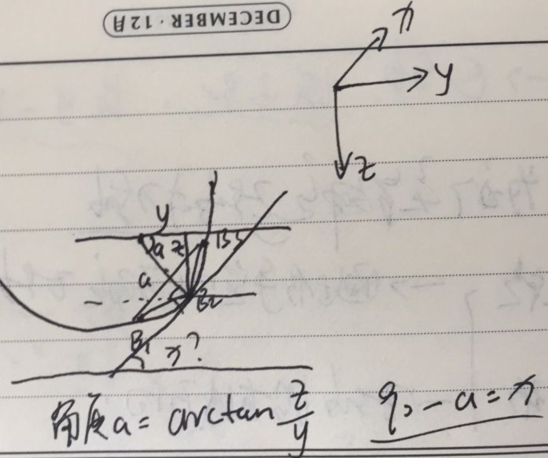通过右手定则得出旋转方向为负，所-x即是我们需要的rz。
展开全文• 最近弄了一个机械臂，怎么用labview控制机械臂旋转的角度，最终达到控制它抓取物体的效果，用的是Elvis 2 这个板子，有没有大佬帮帮忙解答一下啊。我现在连一个舵机也转不起来，不会用Elvis啊。![图片说明]...测试用例
• 国外的机械臂开源项目，电控源码和机械部分都有，适合DIY，国内做这种开源项目的较少，该项目已经成熟，做同类项目的朋友可以参考下，有一定的参考价值，主要是算法部分
• 1、DH坐标下机械臂参数 theta=[pi/10,pi/2,pi/4,-pi/4,pi/4,-pi/8];%关节角度 a=[0, 0.260, 0.025, 0, 0, 0];%连杆长度 d=[0, 0, 0, 0.280, 0, 0.072];%偏移距离 alpha=[pi/2, 0, pi/2, ...%RBR型6轴机械臂 使用...
1、DH坐标下机械臂参数

theta=[pi/10,pi/2,pi/4,-pi/4,pi/4,-pi/8];%关节角度
a=[0, 0.260, 0.025,   0,    0,   0];%连杆长度
d=[0,  0,     0,  0.280,  0, 0.072];%偏移距离

alpha=[pi/2, 0, pi/2, -pi/2 , pi/2, 0];%RBR型6轴机械臂

使用MATLAB的机器人工具箱函数可以画出该机械臂在指定关节角下的姿态，并且给出末端位置和姿态角，代码如下：

​
L1 = Link('d', 0.340,      'a', 0,        'alpha', pi/2 ,'standard' );
L2 = Link('d', 0,          'a', 0.260,   'alpha',   0   ,'standard' );
L3 = Link('d', 0 ,         'a', 0.025,   'alpha', pi/2 ,'standard' );
L4 = Link('d', 0.280,      'a', 0,        'alpha',  -pi/2 ,'standard' );
L5 = Link('d', 0,          'a', 0,        'alpha', pi/2 ,'standard');
L6 = Link('d', 0.072,      'a', 0,        'alpha',   0   ,'standard');

theta=[-pi/2,pi*4/3,pi/4,pi/4,pi/4,-pi/4];%关节角度
STA_MZ204=SerialLink([L1,L2,L3,L4,L5,L6],'name','MZ204-STA');   %SerialLink 类函数
A = fkine(STA_MZ204,theta);//正运动学求解
figure(1),STA_MZ204.teach(theta,'eul');%eul为以欧拉角显示方向

效果如下：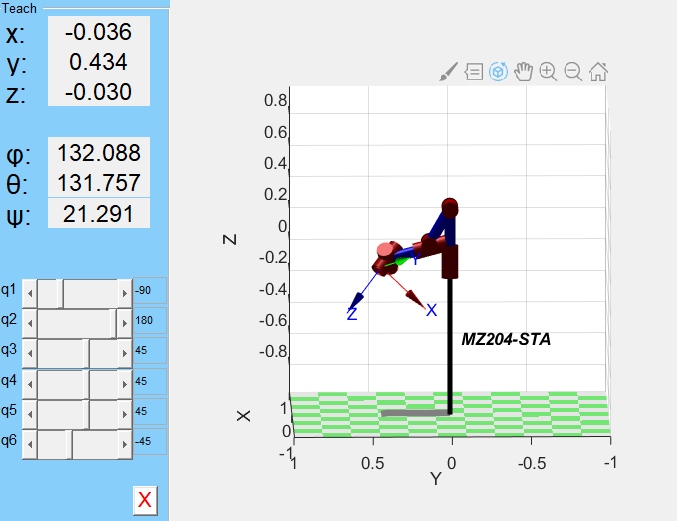二、正运动学求解（MATLAB代码实现）

1、DH坐标系下的T矩阵计算

 T=[cos(theta),-sin(theta)*cos(alpha),sin(theta)*sin(alpha),a*cos(theta);
sin(theta),cos(theta)*cos(alpha),-cos(theta)*sin(alpha),a*sin(theta);
0,sin(alpha),cos(alpha),d;
0,0,0,1];

上面是一个轴的变换矩阵，那么6轴机械臂的变换矩阵T06=T1*T2*T3*T4*T5*T6

2、根据T06矩阵求解末端位置和方向角，方向角为欧拉角（绕ZYZ轴旋转）和方向角（绕XYZ轴旋转）的求解方法不同

末端位置:px=T06(1,4),py=T06(2,4),pz=T06(3,4)

绕ZYZ轴旋转求解：

     r11 = T(1,1);
r21 = T(2,1);
r31 = T(3,1);
r12 = T(1,2);
r22 = T(2,2);
r32 = T(3,2);
r13 = T(1,3);
r23 = T(2,3);
r33 = T(3,3);
px = T(1,4);
py = T(2,4);
pz = T(3,4);
beta_beta = atan2(sqrt(r31*r31+r32*r32),r33) ;%绕Y
beta_alpha = atan2(r23/sin(beta_beta),r13/sin(beta_beta));%先绕Z
beta_yeta = atan2(r32/sin(beta_beta),-r31/sin(beta_beta));%再绕z
pos=[px,py,pz,beta_alpha,beta_beta,beta_yeta];

绕XYZ轴旋转求解：

     nx = T(1,1);
ny = T(2,1);
nz = T(3,1);
%      ox = T(1,2);
%      oy = T(2,2);
oz = T(3,2);
%      ax = T(1,3);
%      ay = T(2,3);
az = T(3,3);
px = T(1,4);
py = T(2,4);
pz = T(3,4);
beta_y = atan2(nz,sqrt(nx*nx+ny*ny)) ;
beta_z = atan2(ny/cos(beta_y),nx/cos(beta_y));
beta_x = atan2(oz/cos(beta_y),az/cos(beta_y));
pos=[px,py,pz,beta_x,beta_y,beta_z];

三、逆运动学求解

逆运动学求解是由给定的末端姿态（位置+方向）求出各个关节的角度，下面将详细叙述求解步骤

1、以ZYZ轴为例，根据末端姿态pos（px,py,pz,theta_a,theta_b,theta_y）求解腕部位置（pwx,pwy,pwz），即三轴交点的位置。

这里要注意的一点是我令d1为0，如果你的机械臂d1不为0的话，你可以将d1等效为0求解，只需要将pz减去d1之后计算即可

 pwx=px-cos(theta_a)*sin(theta_b)*d6;
pwy=py-sin(theta_a)*sin(theta_b)*d6;
pwz=pz-cos(theta_b)*d6;


2、根据腕部位置可以求解出前三个关节的角度，因为腕部的位置是由前三个轴决定的，与后三个轴无关，腕部位置等于第三个轴的末端位置，（令a3=d4，而d4=0）具体可以参考“机器人学建模规划与控制”这本书,不过这种方法只适用于机械臂的a3=0。当a3不为0时，我还是建议使用数学推导，根据具体情况来求解

上面的说法让你有些糊涂的话，我们可以直接用数学推导来说明，当你令d6=0时，机械臂的末端位置便是腕部位置，计算出d6=0时的px,py,pz如下：

px =d4*(cos(theta1)*cos(theta2)*sin(theta3) + cos(theta1)*cos(theta3)*sin(theta2)) + a2*cos(theta1)*cos(theta2) + a3*cos(theta1)*cos(theta2)*cos(theta3) - a3*cos(theta1)*sin(theta2)*sin(theta3)

py =d4*(cos(theta2)*sin(theta1)*sin(theta3) + cos(theta3)*sin(theta1)*sin(theta2)) + a2*cos(theta2)*sin(theta1) + a3*cos(theta2)*cos(theta3)*sin(theta1) - a3*sin(theta1)*sin(theta2)*sin(theta3)

pz =a2*sin(theta2) - d4*(cos(theta2)*cos(theta3) - sin(theta2)*sin(theta3)) + a3*cos(theta2)*sin(theta3) + a3*cos(theta3)*sin(theta2)


从上面的px,py,pz计算结果我们可以发现，他只与theta1,theta2,theta3相关

我们对其进行化简令cos(theta2)=c2,cos(theta2+theta3)=c23得

pwx=px=d4c1s23+c1(a2c2+a3c23)
pwy=py=d4s1s23+s1(a2c2+a3c23)
pwz=pz=a2s2+a3s23-d4c23

从上面可以推出前三个关节的角度theta3,theta2,theta1,主要是一些三角函数推导计算，就不详细说明了，直接上程序

pos2(1)为pwx,pos2(2)为pwy,pos2(3)为pwz
%关节角3求解
m3 = (pos2(1)^2+pos2(2)^2+pos2(3)^2-a(2)^2-a(3)^2-d(4)^2)/(2*a(2))
n3 = sqrt(d(4)^2+a(3)^2)
theta3(1,1) = atan2(m3/n3,sqrt(1-m3^2/n3^2))-atan2(a(3),d(4));
theta3(1,2) = atan2(m3/n3,-sqrt(1-m3^2/n3^2))-atan2(a(3),d(4));

%关节角2求解
m2(1,1:2) = a(2)+a(3)*cos(theta3)+d(4)*sin(theta3)%m2(1)--theta3(1),m2(2)--theta3(2)
n2(1,1:2) = -a(3)*sin(theta3)+d(4)*cos(theta3)%n2(1)--theta3(1),n2(2)--theta3(2)

theta2(1,1:2)=atan2(n2,m2)+atan2(pos2(3),sqrt(n2.*n2+m2.*m2-pos2(3)*pos2(3)));%theta2(1,1)--theta3(1,1),theta2(1,2)--theta3(1,2)
theta2(1,3:4)=atan2(n2,m2)+atan2(pos2(3),-sqrt(n2.*n2+m2.*m2-pos2(3)*pos2(3)));%theta2(1,3)--theta3(1,1),theta2(1,4)--theta3(1,2)
test2=sqrt(n2.*n2+m2.*m2-pos2(3)*pos2(3))
test3=atan2(pos2(3),-sqrt(n2.*n2+m2.*m2-pos2(3)*pos2(3)))
%关节角1求解
theta1(1,1)=atan2(pos2(2),pos2(1));

if(pos2(2)>=0)
theta1(1,2)=atan2(pos2(2),pos2(1))-pi;
else
theta1(1,2)=atan2(pos2(2),pos2(1))+pi;
end

3、根据计算出来的theta1,theta2,theta3,我们可以计算出R03，同时根据位姿我们可以计算出R06，那么就可以求解出R36，

R03是T03=T01*T12*T23的一部分（前三行，三列，表示方向变换部分），同理R06也是如此。

下面给出R03的计算代码

R03(1,1)=cos(theta1).*cos(theta2).*cos(theta3) - cos(theta1).*sin(theta2).*sin(theta3);
R03(2,1)=cos(theta2).*cos(theta3).*sin(theta1) - sin(theta1).*sin(theta2).*sin(theta3);
R03(3,1)=cos(theta2).*sin(theta3) + cos(theta3).*sin(theta2);

R03(1,2)= sin(theta1);
R03(2,2)=-cos(theta1);
R03(3,2)=0;

R03(1,3)=cos(theta1).*cos(theta2).*sin(theta3) + cos(theta1).*cos(theta3).*sin(theta2);
R03(2,3)=cos(theta2).*sin(theta1).*sin(theta3) + cos(theta3).*sin(theta1).*sin(theta2);
R03(3,3)=sin(theta2).*sin(theta3) - cos(theta2).*cos(theta3);

根据末端姿态pos计算R06的代码如下


R06(1,1)=cos(pos(6))*cos(pos(5))*cos(pos(4))-sin(pos(4))*sin(pos(6));
R06(2,1)=cos(pos(6))*cos(pos(5))*sin(pos(4))+cos(pos(4))*sin(pos(6));
R06(3,1)=-sin(pos(5))*cos(pos(6));

R06(1,2)=-sin(pos(6))*cos(pos(5))*cos(pos(4))-cos(pos(6))*sin(pos(4));
R06(2,2)=-sin(pos(6))*cos(pos(5))*sin(pos(4))+cos(pos(6))*cos(pos(4));
R06(3,2)=sin(pos(5))*sin(pos(6));

R06(1,3)=cos(pos(4))*sin(pos(5));
R06(2,3)=sin(pos(4))*sin(pos(5));
R06(3,3)=cos(pos(5));


因为R06=R03*R36;则R36=INV(R03)*R06,在MATLAB里可以用R36=R03/R06来计算。

接下来讲如何根据R36求解theta4,theta5,theta6

首先我们看一下R36的数学表达式

     R36(1,1)=cos(theta4)*cos(theta5)*cos(theta6) - sin(theta4)*sin(theta6);
R36(2,1)=cos(theta4)*sin(theta6) + cos(theta5)*cos(theta6)*sin(theta4);
R36(3,1)= -cos(theta6)*sin(theta5);

R36(1,2)= - cos(theta6)*sin(theta4) - cos(theta4)*cos(theta5)*sin(theta6);
R36(2,2)= cos(theta4)*cos(theta6) - cos(theta5)*sin(theta4)*sin(theta6);
R36(3,2)= sin(theta5)*sin(theta6);

R36(1,3)=cos(theta4)*sin(theta5);
R36(2,3)=sin(theta4)*sin(theta5);
R36(3,3)=cos(theta5);

根据R36的表达式我们便可以求出theta4,theta5,theta6

具体代码如下：

    %theta5(0,pi),
theta4(1,i)=atan2(R36(2,3),R36(1,3));
theta5(1,i)=atan2(sqrt(R36(2,3)^2+R36(1,3)^2),R36(3,3));
theta6(1,i)=atan2(R36(3,2),-R36(3,1));

%theta5(-pi,0),
theta4(2,i)=atan2(-R36(2,3),-R36(1,3));
theta5(2,i)=atan2(-sqrt(R36(2,3)^2+R36(1,3)^2),R36(3,3));
theta6(2,i)=atan2(-R36(3,2),R36(3,1));

4、注意theta1,theta2,theta3的解一共有四组，分别是

//theta1(1,1)，theta2(1,1)，theta3(1,1)
//theta1(1,1)，theta2(1,2)，theta3(1,2)
//theta1(1,2)，theta2(1,3)，theta3(1,1)
//theta1(1,2)，theta2(1,4)，theta3(1,2)
每一组解对应的theta4,theta5,theta6有两组解

所以逆运动学的解一共有8组

展开全文MATLAB代码实现
• noname:: 6 axis, RRRRRR, stdDH, slowRNE +---+-----------+-----------+-----------+-----------+-----------+ | j | theta | d | a | alpha | offset | +---+-----------+-----------+-----------+-----------+-...
机器人工具箱常用函数
Link类
Link 对象包括连杆的各种属性：运动学参数、惯性张量、电机、传递矩阵等
Link 的类函数：
信息/显示方式：
display : 显示连杆参数表格
dyn : 显示动力学参数
type: 关节类型:‘R’或者’P’
转换方式：
char : 转化为字符串
运算方式：
A ：关节传动矩阵
friction : 摩擦力
nofriction : 摩擦为0
测试方式：
islimit：检测关节变量是否超出范围
isrevolute : 检测关节是否为转动关节
isprismatic : 检测关节是否为移动关节
issym: 检测关节和连杆是否有符号参数
Link 的类属性（读/写）：
运动学：
theta: 关节角
d: 连杆偏移量
a: 连杆长度
alpha:连杆转角
mdh: 默认0，SDH；1，MDH
offset:关节变量偏移量
qlim:关节变量范围[min max]
动力学：
m: 质量
r: 质心
I: 惯性张量
B: 粘性摩擦
Tc: 静摩擦
G: 减速比
Jm: 转子惯量
Seriallink 类
类函数比较多，包括显示机器人、动力学、逆动力学、雅可比等
Seriallink 的类函数：
显示/画图方式：
animate: 动画机器人模型
display: 显示连杆参数表格
dyn: 显示动力学参数
edit: 显示和编辑运动学与动力学参数
getpos: 获取机器人图形位置
plot: 显示机器人模型
plot3d: 显示机器人3d模型
teach: 驱动机器人模型
显示/画图方式：
islimit: 检测机器人是否超出范围
isconfig: 检测机器人关节结构属性
issym: 检测关节和连杆是否有符号参数
isprismatic: 检测是否移动关节
isrevolute: 检测是否转动关节
isspherical: 检测是否为球关节
转换方式：
char : 转化为字符串
sym: 转化为符号参数
todegrees: 关节角转化为角度
toradians: 关节角转化为弧度
选项
‘name’，NAME: 设置机器人名字属性为NAME
‘manufacturer’,MANUF : 设置机器人制造者的名字为MANUF
‘comment’,COMMENT: 设置机器人注释为COMMENT
‘base’,T: 设置基坐标系矩阵属性为T
‘tool’,T: 设置工具坐标系矩阵属性为T
‘gravity,G’: 设置重力矢量属性为G
‘plotpt’,P: 为.plot()设置默认选项为P
‘plotpt3d’,P: 为.plot3d()设置默认选项为P
‘nofast’: 不使用
1、SDH建模仿真：
L1 = Link('d', 0, 'a', 160, 'alpha', -pi/2);
L2 = Link('d', 0, 'a', 580, 'alpha', 0,'offset',-pi/2);
L3 = Link('d', 0, 'a', 200, 'alpha', -pi/2);
L4 = Link('d', 640, 'a', 0, 'alpha', pi/2);
L5 = Link('d', 228, 'a', 0, 'alpha', -pi/2);
L6 = Link('d', 0, 'a', 0, 'alpha', 0);
robot=SerialLink([L1,L2,L3,L4,L5,L6]);   %SerialLink 类函数
robot.display();  %Link 类函数
theta=[0 0 0 0 0 0];
robot.plot(theta);   %SerialLink 类函数

运行结果：
robot =

noname:: 6 axis, RRRRRR, stdDH, slowRNE
+---+-----------+-----------+-----------+-----------+-----------+
| j |     theta |         d |         a |     alpha |    offset |
+---+-----------+-----------+-----------+-----------+-----------+
|  1|         q1|          0|        160|    -1.5708|          0|
|  2|         q2|          0|        580|          0|    -1.5708|
|  3|         q3|          0|        200|    -1.5708|          0|
|  4|         q4|        640|          0|     1.5708|          0|
|  5|         q5|        228|          0|    -1.5708|          0|
|  6|         q6|          0|          0|          0|          0|
+---+-----------+-----------+-----------+-----------+-----------+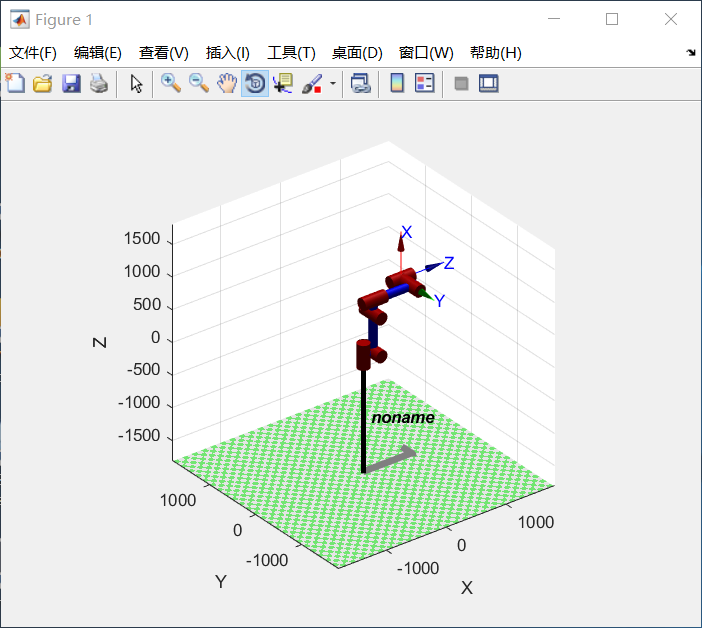2、MDH建模仿真：
L1 =  Link([ 0,    450,       0,          0,     0,     0], 'modified');
L2 =  Link([ 0,     0,       160,       -pi/2,   0, -pi/2], 'modified');
L3 =  Link([ 0,     0,       580,         0,     0,     0], 'modified');
L4 =  Link([ 0,    640,      200,       -pi/2,   0,     0], 'modified');
L5 =  Link([ 0,     0,        0,         pi/2,   0,     0], 'modified');
L6 =  Link([ 0,    228,       0,        -pi/2,   0,     0], 'modified');
robot=SerialLink([L1,L2,L3,L4,L5,L6]);   %SerialLink 类函数
robot.display();  %Link 类函数
theta=[0 0 0 0 0 0];
robot.plot(theta);   %SerialLink 类函数

robot =

noname:: 6 axis, RRRRRR, modDH, slowRNE
+---+-----------+-----------+-----------+-----------+-----------+
| j |     theta |         d |         a |     alpha |    offset |
+---+-----------+-----------+-----------+-----------+-----------+
|  1|         q1|        450|          0|          0|          0|
|  2|         q2|          0|        160|    -1.5708|    -1.5708|
|  3|         q3|          0|        580|          0|          0|
|  4|         q4|        640|        200|    -1.5708|          0|
|  5|         q5|          0|          0|     1.5708|          0|
|  6|         q6|        228|          0|    -1.5708|          0|
+---+-----------+-----------+-----------+-----------+-----------+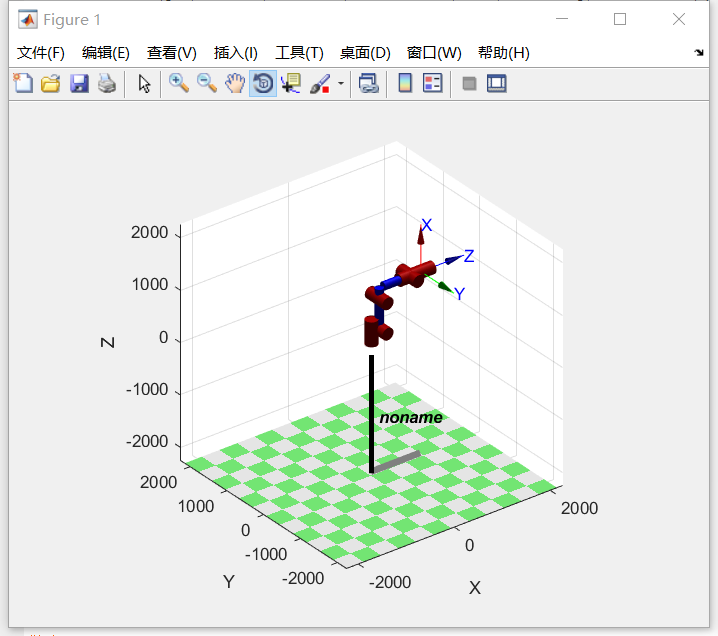展开全文• DeepPoseRobot，DeepPoseKit的实现 这是和的改编版，用于预测机器人关节角度。 可视化使用 。 使用PixelLib将机器人与背景隔离，然后使用DeepPoseKit模型预测机器人的关键点位置。 安装 这需要进行分割和姿势估计...Python
• Python3.7.3(Win10)解释环境下载 python IDE编辑器Sublime Text 3 (3211) 简介 Sublime Text 是一个文本编辑器（收费软件，可以无限期试用，但是会有激活提示弹窗），同时也是一个先进的代码编辑器。...windows
• 机械臂的逆运动学问题就是由给定的末端执行器位置和方向，确定机械臂各个关节变量的值。机械臂的求解方法可以分为两大类：数值解和解析解（封闭解）。  数值解和解析解有各自的特点，商用的机械臂一般都会采用解析...
• 关注微信公众号二进制人工智能并回复robot，获取MATLAB机器人工具箱 1 在平面上画字母C mdl_puma560 aplha=pi/4:pi/40:2*pi-pi/4; k=inf; % 斜面斜率k r=1; letterShrink=0.2; %缩放倍数 letterTranslX=0.4;...if
• ## 六轴机械臂算法-引导篇

万次阅读 多人点赞 2018-06-02 12:41:09
最近一直在研究 6轴机械臂算法，整理出了如下几个计算六轴机械臂正解和逆解的关键点： 01_机器人坐标系和关节的说明 02_算法坐标系的建立 03_D-H参数表的建立 04_FK（正解）算法 05_Matlab辅助计算FK（正解） ...
• 如果机械臂后三个相交在一点（这使手臂末端在指定的位置可以到达任意角度，所以常常这样设计），则轴6到0的向量和4到0的向量是相同的（即，xyz相同）。求各角度的法则就是使它们各自分离。 我们把iii到i−1...
• 输出总共12只脚，所以最高6轴联动。 输入有5只脚，可以接行程开关，原点开关，报警信号等 例程1学会这些就够了。 再学例程2，要用到计算机内部的高精度定时器，步进电机或伺服电机对脉冲要求较高，普能定时器精度...
• 因此，我用lego的EV3砖块和伺服器构建了一个6轴机械臂。 现在我需要软件来控制它。 要将手臂的尖端移动到所需位置，我只想在手臂可触及范围内的三维空间中指定该点。 人工智能应该为我完成所有艰苦的工作。 开始 我...JavaScript
• 用zyz Euler 求θ4,θ5,θ6\theta_4, \theta_5, \theta_6θ4​,θ5​,θ6​ 这里zyz 是对着一个绝对的frame 依次绕zyz转动，而DH是按关节顺序依次相对于当前关节的frame绕z转动的。因此，要注意用zyz计算出来的...
• Faze4是可完全3d打印的小型开源6轴机械臂。 它在功能和美学上都与工业机械手臂类似，但主要用于研究，教育和任何有兴趣制造自己的机械手臂的人。 该手臂与其他DIY手臂分开的主要“卖点”是： <1000美元便宜。 ...C++
• 6轴机械臂，3个主轴（基本轴）用以保证末端执行器达到工作空间的任意位置，3个次轴（腕部轴）用以返回实现末端执行器的任意空间姿态。 2 坐标系 大部分商用工业机器人系统中，均可使用关节坐标系、直角坐标系、...
• 下一步进行机械手臂的程序编写 程序只是进行简单的点位运动，实现抓取功能 程序控制的点位表 输入点位 点位描述 输出点位 点位描述 DI5 夹取完成 DO5 夹取物料 DI6 物料到位 DO6 放下物料 DI7 ...
• 轴机械臂从下位机（arduino）+上位机（ros+moveit） 目录 一、机械臂的硬件 1、机械部分 2、电控部分 二、机械臂的下位机搭建 1、控制器接线 2、注意事项 三、机械臂的上位机搭建 1、ROS环境的搭建 2...ROS gazebo
• 博主最近在学一本叫做《A Mathematical Introduction to Robotic Manipulation》的机器人学教材，并且在学习之...Analyze workspaces of 4 axis robot and 6 axis robot, find the intersection area firstly. Com...机器人学 MATLAB
• 这款6轴桌面机械臂由具有Web界面的Raspberry Pi控制 Web界面来控制机械臂 Python程序使用Flask Web服务器托管一个带有六个滑块的网站，以彼此独立地控制每个伺服电机。 下图显示了易于使用的Web界面。 分步指南 要...HTML
• 开源算法六轴机械臂 自从为多个开放源代码项目提供匿名帐户以来已经过去了6个月，从我修正一些错字开始，但是现在我每周为开放源代码项目贡献约20个小时，我很喜欢。 当我向没有编写代码的朋友解释我的所作所为时...java python 编程语言 人工智能 深度学习
• 利用halcon软件，6轴机械臂进行抓取螺丝的程序可结合halcon实例来进行手眼标定后的抓取任务。具体程序放在
• 今天，我将向您介绍MPU-6050（加速度计+陀螺仪）传感器模块，以及如何通过它控制由微型伺服电机制成的简单2轴机械臂。 InvenSense MPU-6050是一款低成本，高精度，六自由度（DOF）的惯性测量单元（IMU ）。IMU可以...
• 硬件 三菱fx3n控制器 点位表 ...do6 y5 di6 x6 do7 y6 di7 y7 di8 plc输入 输入描述 plc输出 输出描述 x10 夹取气缸到位 y10 夹取气缸 x11 放料气缸到位 y11 放料气缸 x12 物料到位
• FreeCAD中的机械臂模型是由.wrl和.csv文件两部分构成的，在...其中.wrl文件主要是用来定义机械臂的外形特征和几何连接关系，.csv文件是用来保存6轴机械臂的DH参数和转角及速度约束。文档中具体介绍如何建立这两个文件。
• ## 机械臂运动学逆解

千次阅读 多人点赞 2019-07-16 16:32:49
相信小伙伴都在淘宝上买了这个手臂来玩耍，但是这手跟工业传统的6轴机械臂那差了不是一点半点。找寻了半天也没有找到现成的DH模型和运动学逆解。所有就自己使用几何法建立了模型并给出运动学逆解。最后的控制效果...